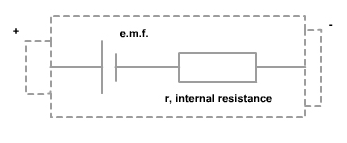# EMF of a cell

The electromotive force (ξ) or e.m.f. is the energy provided by a cell or battery per coulomb of charge passing through it, it is measured in volts (V). It is equal to the potential difference across the terminals of the cell when no current is flowing. When current flows round a circuit energy is transformed in both the external resistor but also in the cell itself. All cells have a resistance of their own and we call this the internal resistance of the cell. The voltage produced by the cell is called the electromotive force or e.m.f for short and this produces a p.d across the cell and across the external resistor.

ξ = E / Q

• ξ= electromotive force in volts, V
• E = energy in joules, J
• Q = charge in coulombs, C
•ξ = V + Ir = IR + Ir

Both emf and pd are measured in volts, V, as they describe how much energy is put in or taken out per coulomb of charge passing through that section of the circuit.

### Difference between EMF and voltage

EMF is the voltage developed between two terminals of a battery or source in the absence of electric current. In otherhand Voltage is the potential difference developed between the two electrode potentials of a battery under any conditions.